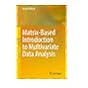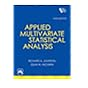Normal view MARC view ISBD view

# Matrix-based introduction to multivariate data analysis.

Material type:TextPublisher: New York, NY : Springer Berlin Heidelberg, 2016Description: xi, 301 pages, UKP 79.99 ill.; 24 cms.Content type: text Media type: unmediated Carrier type: volumeISBN: 9789811023408(hbk).
Contents:
Part 1. Elementary Statistics with Matrices -- 1 Introduction to Matrix Operations -- 2 Intra-variable Statistics -- 3 Inter-variable Statistics -- Part 2. Least Squares Procedures -- 4 Regression Analysis -- 5 Principal Component Analysis (Part 1) -- 6 Principal Component Analysis 2 (Part 2) -- 7 Cluster Analysis -- Part 3. Maximum Likelihood Procedures -- 8 Maximum Likelihood and Normal Distributions -- 9 Path Analysis -- 10 Confirmatory Factor Analysis -- 11 Structural Equation Modeling -- 12 Exploratory Factor Analysis -- Part 4. Miscellaneous Procedures -- 13 Rotation Techniques -- 14 Canonical Correlation and Multiple Correspondence Analyses -- 15 Discriminant Analysis -- 16 Multidimensional Scaling -- Appendices -- A1 Geometric Understanding of Matrices and Vectors -- A2 Decomposition of Sums of Squares -- A3 Singular Value Decomposition (SVD) -- A4 Matrix Computation Using SVD -- A5 Supplements for Probability Densities and Likelihoods -- A6 Iterative Algorithms -- References -- Index.
Summary: This book enables readers who may not be familiar with matrices to understand a variety of multivariate analysis procedures in matrix forms. Another feature of the book is that it emphasizes what model underlies a procedure and what objective function is optimized for fitting the model to data. The author believes that the matrix-based learning of such models and objective functions is the fastest way to comprehend multivariate data analysis. The text is arranged so that readers can intuitively capture the purposes for which multivariate analysis procedures are utilized: plain explanations of the purposes with numerical examples precede mathematical descriptions in almost every chapter. This volume is appropriate for undergraduate students who already have studied introductory statistics. Graduate students and researchers who are not familiar with matrix-intensive formulations of multivariate data analysis will also find the book useful, as it is based on modern matrix formulations with a special emphasis on singular value decomposition among theorems in matrix algebra. The book begins with an explanation of fundamental matrix operations and the matrix expressions of elementary statistics, followed by the introduction of popular multivariate procedures with advancing levels of matrix algebra chapter by chapter. This organization of the book allows readers without knowledge of matrices to deepen their understanding of multivariate data analysis.
List(s) this item appears in: 2018-06-22
Item type Current location Call number Status Date due Barcode Item holds
Book Chennai Mathematical Institute
General Stacks
519.522 ADA (Browse shelf) Available 10382
Total holds: 0
##### Browsing Chennai Mathematical Institute Shelves , Shelving location: General Stacks Close shelf browser519.52 BOS U-statistics, mm-estimators and resampling/ TRIM 75 519.52 FER A course in large sample theory / 519.52 LEH Elements of large-sample theory / 519.522 ADA Matrix-based introduction to multivariate data analysis. 519.535 AGR Categorical data analysis / 519.535 AND An introduction to multivariate statistical analysis / 519.535 JOH Applied multivariate statistical analysis /

Includes references and index.

Part 1. Elementary Statistics with Matrices -- 1 Introduction to Matrix Operations -- 2 Intra-variable Statistics -- 3 Inter-variable Statistics -- Part 2. Least Squares Procedures -- 4 Regression Analysis -- 5 Principal Component Analysis (Part 1) -- 6 Principal Component Analysis 2 (Part 2) -- 7 Cluster Analysis -- Part 3. Maximum Likelihood Procedures -- 8 Maximum Likelihood and Normal Distributions -- 9 Path Analysis -- 10 Confirmatory Factor Analysis -- 11 Structural Equation Modeling -- 12 Exploratory Factor Analysis -- Part 4. Miscellaneous Procedures -- 13 Rotation Techniques -- 14 Canonical Correlation and Multiple Correspondence Analyses -- 15 Discriminant Analysis -- 16 Multidimensional Scaling -- Appendices -- A1 Geometric Understanding of Matrices and Vectors -- A2 Decomposition of Sums of Squares -- A3 Singular Value Decomposition (SVD) -- A4 Matrix Computation Using SVD -- A5 Supplements for Probability Densities and Likelihoods -- A6 Iterative Algorithms -- References -- Index.

This book enables readers who may not be familiar with matrices to understand a variety of multivariate analysis procedures in matrix forms. Another feature of the book is that it emphasizes what model underlies a procedure and what objective function is optimized for fitting the model to data. The author believes that the matrix-based learning of such models and objective functions is the fastest way to comprehend multivariate data analysis. The text is arranged so that readers can intuitively capture the purposes for which multivariate analysis procedures are utilized: plain explanations of the purposes with numerical examples precede mathematical descriptions in almost every chapter. This volume is appropriate for undergraduate students who already have studied introductory statistics. Graduate students and researchers who are not familiar with matrix-intensive formulations of multivariate data analysis will also find the book useful, as it is based on modern matrix formulations with a special emphasis on singular value decomposition among theorems in matrix algebra. The book begins with an explanation of fundamental matrix operations and the matrix expressions of elementary statistics, followed by the introduction of popular multivariate procedures with advancing levels of matrix algebra chapter by chapter. This organization of the book allows readers without knowledge of matrices to deepen their understanding of multivariate data analysis.